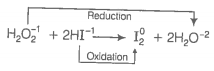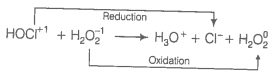Consider the reactions

(i) ${\mathrm{H}}_{2}{\mathrm{O}}_{2}+2\mathrm{HI}\to {\mathrm{I}}_{2}+2{\mathrm{H}}_{2}\mathrm{O}$

(ii) $\mathrm{HOCl}+{\mathrm{H}}_{2}{\mathrm{O}}_{2}\to {\mathrm{H}}_{3}{\mathrm{O}}^{+}+{\mathrm{Cl}}^{-}+{\mathrm{O}}_{2}$

Which of the following statements is correct about H2O2 with reference to these reactions? Hydrogen peroxide is ______.

1.  an oxidising agent in both (i) and (ii)

2.  an oxidisng agent in (i) and reducing agent in (ii)

3.  a reducing agent in (i) and oxidising agent in (ii)

4.  a reducing agent in both (i) and (ii)

Hint: Loss of electrons oxidation and gain of electrons reduction
In the first reaction, oxidation of I is change from -1 to 0. HI undergo oxidation reaction.Thus, here H2O2 oxidises HI into I2 hence, it behaves as oxidising agent.
In the second reaction, oxidation of Cl is change from +1 to -1. HOCl undergo reduction reaction.Here, H2O2 reduces to HOCl to Cl-1, thus, it acts as reducing agent.